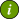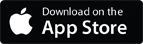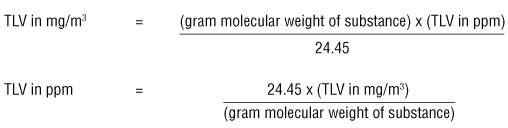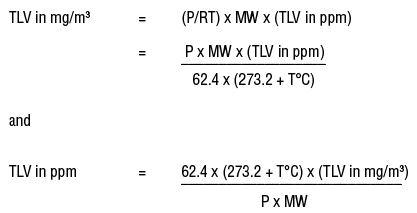### OSH Answers Fact SheetsEasy-to-read, question-and-answer fact sheets covering a wide range of workplace health and safety topics, from hazards to diseases to ergonomics to workplace promotion. MORE ABOUT >Search all fact sheets:

Type a word, a phrase, or ask a question

# Converting Occupational Exposure Limits from mg/m3 to ppm

## When can I convert mg/m3 to ppm?

Occupational exposure limits (OELs, TLVs, PELs, etc.) can be expressed in parts per million (ppm) only if the substance exists as a gas or vapour at normal room temperature and pressure. This is why exposure limits are usually expressed in mg/m3. However, some OELs may be expressed in units such as fibres/cc (e.g., for asbestos). OELs for metals, salts and other compounds that do not form vapours at room temperature and pressure are expressed in mg/m3 only.

## What is the usual way of converting mg/m3 to ppm?

The ACGIH booklet "Threshold Limit Values (TLVs™) for Chemical Substances and Physical Agents and Biological Exposure Indices (BEIs™)" uses the formulas:These formulas can be used when measurements are taken at 25°C and the air pressure is 760 torr (= 1 atmosphere or 760 mm Hg).

## What is gram molecular weight?

Gram molecular weight is the molecular weight (MW) of a substance expressed in grams. For example, the gram molecular weight for toluene is 92.13 g. since the molecular weight is 92.13. A gram molecular weight is also called a gram mole.

## How do I convert mg/m3 to ppm at different temperatures and pressures?

The number 24.45 in the equations above is the volume (liters) of a mole (gram molecular weight) of a gas or vapour when the pressure is at 1 atmosphere (760 torr or 760 mm Hg) and at 25°C.

To convert mg/m3 to ppm at other temperatures and pressures, one must calculate the volume of 1 gram molecular weight of an airborne contaminant (e.g. 92.13 grams of toluene) by using the formula:

V = (RT/ P)

where R is the ideal gas constant; T is the temperature in  kelvins (273.16 + T°C); and P is the pressure in mm Hg. This information can be substituted in the formulas for converting between mg/m3 and ppm.where the value of R is 62.4 L torr/mol K when the temperature (T) is in kelvins (K), (K =273.16 + T°C), the pressure is expressed in units of mm Hg (1 mmHg = 1 torr), and the volume is in liters. The gas constant can have different values if the temperature and pressure are expressed in different units from those in the above equation.

Document last updated on November 1, 2016